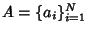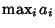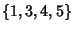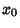## Maximum

The largest value of a set, function, etc. The maximum value of a set of elementsis denotedor, and is equal to the last element of a sorted (i.e., ordered) version of. For example, given the set, the sorted version is, so the maximum is 5. The maximum and Minimum are the simplest Order Statistics.A continuous Function may assume a maximum at a single point or may have maxima at a number of points. A Global Maximum of a Function is the largest value in the entire Range of the Function, and a Local Maximum is the largest value in some local neighborhood.

For a functionwhich is Continuous at a point, a Necessary but not Sufficient condition forto have a Relative Maximum atis thatbe a Critical Point (i.e.,is either not Differentiable atoris a Stationary Point, in which case).

The First Derivative Test can be applied to Continuous Functions to distinguish maxima from Minima. For twice differentiable functions of one variable,, or of two variables,, the Second Derivative Test can sometimes also identify the nature of an Extremum. For a function, the Extremum Test succeeds under more general conditions than the Second Derivative Test.

See also Critical Point, Extremum, Extremum Test, First Derivative Test, Global Maximum, Inflection Point, Local Maximum, Midrange, Minimum, Order Statistic, Saddle Point (Function), Second Derivative Test, Stationary Point

References

Abramowitz, M. and Stegun, C. A. (Eds.). Handbook of Mathematical Functions with Formulas, Graphs, and Mathematical Tables, 9th printing. New York: Dover, p. 14, 1972.

Press, W. H.; Flannery, B. P.; Teukolsky, S. A.; and Vetterling, W. T. Minimization or Maximization of Functions.'' Ch. 10 in Numerical Recipes in FORTRAN: The Art of Scientific Computing, 2nd ed. Cambridge, England: Cambridge University Press, pp. 387-448, 1992.

Tikhomirov, V. M. Stories About Maxima and Minima. Providence, RI: Amer. Math. Soc., 1991.